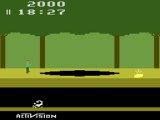Sam Trenholme's webpage
Support this website# A 4096-byte jungle

## June 6 2013

In 1982, the video game to have was Activision’s Pitfall, which featured one Pitfall Harry running, jumping, climbing, using vines and tunnels to travel through a jungle seeking treasure.

Even though the video game allowed Harry to travel through a huge jungle with some 255 different locations, and included then cutting edge graphics including snakes, scorpions, alligators, and four different type of treasures, the entire game fit in only 4,096 bytes.

Let me repeat that: 4,096 bytes. Not kilobytes. Not megabytes. Just bytes. 4,096 bytes is the equivalent of one—possibly two—pages of written text.

==Storing the jungle==

As mentioned above, this 4,096-byte game stored some 255 different locations to explore in the jungle. In that little space, it was not possible to have even a 256-byte look-up table with a map of the jungle. Instead, David Crane (Pitfall’s developer) used a pseudo-random number generator.

In particular there are two pieces of code: One piece that uses the random number generator to calculate what the screen to the right of a given screen looks like, and another piece of code, the inverse function which calculates what happens when one travels left.

At the beginning of the game, Pitfall Harry starts in location “c4”, which means “This room has a ladder in the middle, a single unmoving log, and a wall on the right hand side underground.”

Immeditely to the left of location “c4” is “e2”, which has a scorpion underground and a crocodile pit in the jungle.

==Calculating a location==

The code that takes the number like “c4” (which is 196 in normal decimal numbers) and converts it in to, as in this example, the number “e2” (226) looks like this in the original 6502 Pitfall code:

LDA \$81 ;# A5 81
ASL     ;# 0A
EOR \$81 ;# 45 81
ASL     ;# 0A
EOR \$81 ;# 45 81
ASL     ;# 0A
ASL     ;# 0A
ROL     ;# 2A
EOR \$81 ;# 45 81
LSR     ;# 4A
ROR \$81 ;# 66 81

Translated directly in to C, the 6502 looks like this:

uint8_t left_random(uint8_t random) {
uint8_t a, c, t; // a: accumulator c: carry t: temp
a = random;                                             // lda random
c = a >> 7; a <<= 1;                                    // asl
a ^= random;                                            // eor random
c = a >> 7; a <<= 1;                                    // asl
a ^= random;                                            // eor random
c = a >> 7; a <<= 1;                                    // asl
c = a >> 7; a <<= 1;                                    // asl
t = a; a <<= 1; a |= (c & 1); c = t >> 7;               // rol
a ^= random;                                            // eor random
c = a & 1; a >>= 1;                                     // lsr
t = random; random >>= 1; random |= (c << 7); c = t & 1;// ror random
return random;
}

This more concise code functions identically:

uint8_t left_random(uint8_t random) {
uint8_t t; // temp
t = (random >> 6) ^ (random >> 5) ^ (random >> 4) ^ random;
random >>= 1;
random |= t << 7;
return random;
}

To allow the player to move either left or right, David Crane added this code to calculate what happens when the player moves to the right. This code, for example, takes “e2” and converts it back in to “c4”:

LDA \$81 ;# A5 81
ASL     ;# 0A
EOR \$81 ;# 45 81
ASL     ;# 0A
EOR \$81 ;# 45 81
ASL     ;# 0A
ASL     ;# 0A
EOR \$81 ;# 45 81
ASL     ;# 0A
ROL \$81 ;# 26 81

The “ROL” operation looks like this when directly translated in to C:

t = random; random <<= 1; random |= (c & 1); c = t >> 7;// rol random

And the entire “move one location right” code looks like this in C:

uint8_t right_random(uint8_t random) {
uint8_t t; // temp
t = (random >> 7) ^ (random >> 5) ^ (random >> 4) ^ (random >> 3);
random <<= 1;
random |= (t & 1);
return random;
}

==How does this code work?==

This code allows 255 different locations in Pitfall’s jungle to be represented in only 31 bytes of code. This 224-byte savings was very significant when an entire game had to fit in 4,096 bytes.

The code is a classic pseudo-random number generator called a linear feedback shift register (LFSR). I will discuss LFSRs more, and look at possible variants in Pitfall’s LFSR in a future blog entry.